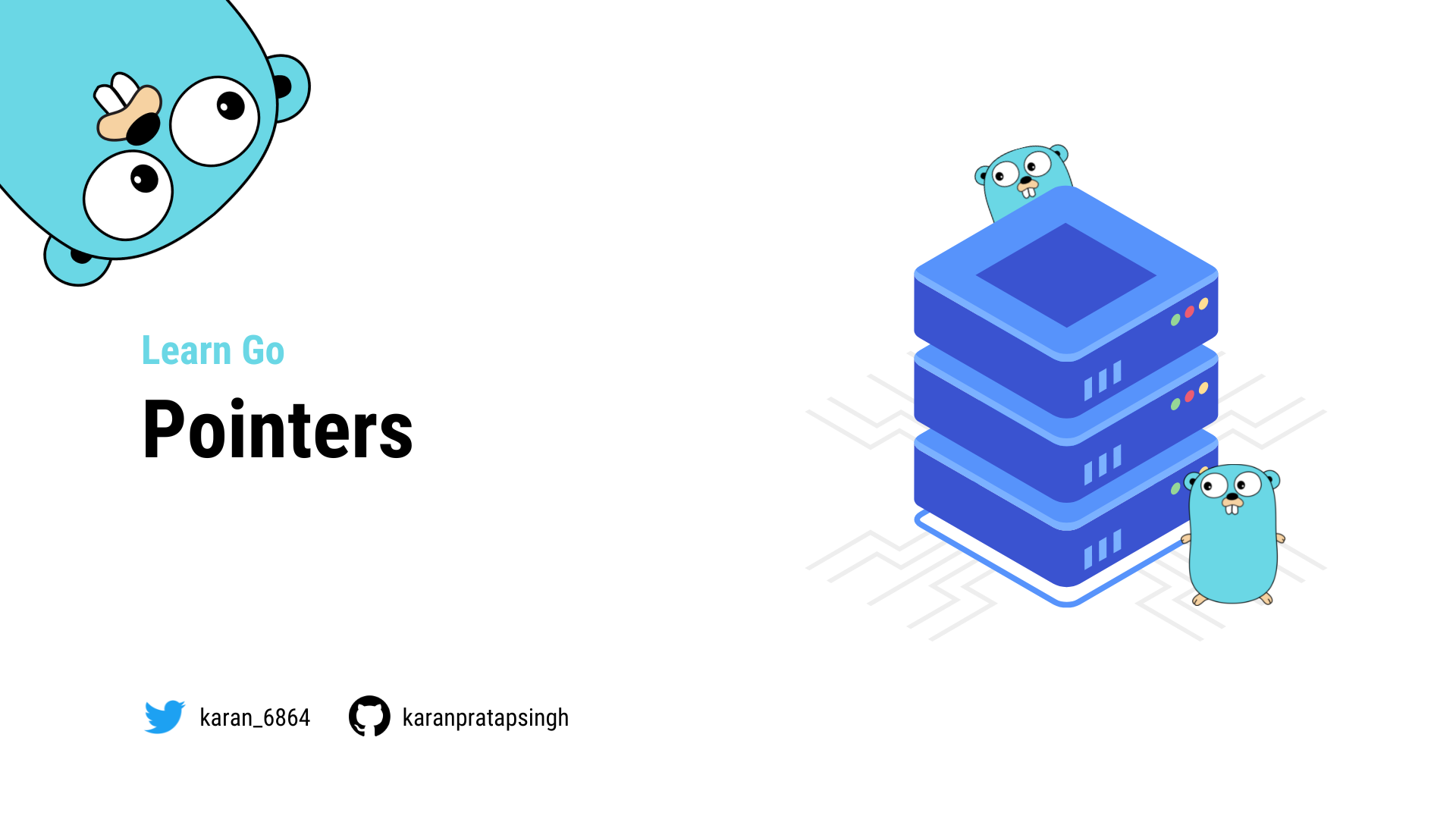# Pointers

Authors
•Name
Karan Pratap Singh
@karan_6864In this tutorial, we will discuss pointers. So what are Pointers?

Simply defined, a Pointer is a variable that is used to store the memory address of another variable.It can be used like this:

``````var x *T
``````

Where `T` is the type such as `int`, `string`, `float`, and so on.

Let's try a simple example and see it in action.

``````package main

import "fmt"

func main() {
var p *int

fmt.Println(p)
}
``````
``````\$ go run main.go
nil
``````

Hmm, this prints `nil`, but what is `nil`?

So nil is a predeclared identifier in Go that represents zero value for pointers, interfaces, channels, maps, and slices.

This is just like what we learned in the variables and datatypes section, where we saw that uninitialized `int` has a zero value of 0, a `bool` has false, and so on.

Okay, now let's assign a value to the pointer.

``````package main

import "fmt"

func main() {
a := 10

var p *int = &a

}
``````

We use the `&` ampersand operator to refer to a variable's memory address.

``````\$ go run main.go
0xc0000b8000
``````

This must be the value of the memory address of the variable `a`.

## Dereferencing

We can also use the `*` asterisk operator to retrieve the value stored in the variable that the pointer points to. This is also called dereferencing.

For example, we can access the value of the variable `a` through the pointer `p` using that `*` asterisk operator.

``````package main

import "fmt"

func main() {
a := 10

var p *int = &a

fmt.Println("value:", *p)
}
``````
``````\$ go run main.go
value: 10
``````

We can not only access it but change it as well through the pointer.

``````package main

import "fmt"

func main() {
a := 10

var p *int = &a

fmt.Println("before", a)

*p = 20
fmt.Println("after:", a)
}
``````
``````\$ go run main.go
before 10
after: 20
``````

I think this is pretty neat!

## Pointers as function args

Pointers can also be used as arguments for a function when we need to pass some data by reference.

Here's an example:

``````myFunction(&a)
...

func myFunction(ptr *int) {}
``````

## New function

There's also another way to initialize a pointer. We can use the `new` function which takes a type as an argument, allocates enough memory to accommodate a value of that type, and returns a pointer to it.

Here's an example:

``````package main

import "fmt"

func main() {
p := new(int)
*p = 100

fmt.Println("value", *p)
}
``````
``````\$ go run main.go
value 100
``````

## Pointer to a Pointer

Here's an interesting idea...can we create a pointer to a pointer? The answer is yes! Yes, we can.

``````package main

import "fmt"

func main() {
p := new(int)
*p = 100

p1 := &p

fmt.Println("P value", *p, " address", p)
fmt.Println("P1 value", *p1, " address", p)

fmt.Println("Dereferenced value", **p1)
}
``````
``````\$ go run main.go
Dereferenced value 100
``````

Notice how the value of `p1` matches the address of `p`.

Also, it is important to know that pointers in Go do not support pointer arithmetic like in C or C++.

``````	p1 := p * 2 // Compiler Error: invalid operation
``````

However, we can compare two pointers of the same type for equality using a `==` operator.

``````p := &a
p1 := &a

fmt.Println(p == p1)
``````

## But Why?

This brings us to the million-dollar question, why do we need pointers?

Well, there's no definite answer for that, and pointers are just another useful feature that helps us mutate our data efficiently without copying a large amount of data.

Lastly, I will add that if you are coming from a language with no notion of pointers, don't panic and try to form a mental model of how pointers work.